# What do you understand by Intrinsic Semiconductor and Extrinsic Semiconductor ?

## Intrinsic Semiconductor

A semiconductor in an extremely pure form is known as an intrinsic semiconductor.

In an intrinsic semiconductor, even at room temperature, hole-electron pairs are created.

When electric field is applied across an intrinsic semiconductor, the current conduction take place by free electrons and holes as shown in fig.1.

The free electrons are produced due to the breaking up of some co-valent bonds by thermal energy. At the same time holes are created in the covalent-bonds.

Under the influence of electric field, conduction takes place by both free electrons and holes.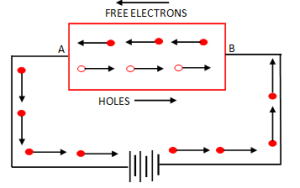Fig.1

Therefore,the total current inside the semiconductor is the sum of currents due to free electrons and holes.

It can be noted that current in the external wire is fully due to electrons.

Referring to fig.1, holes being positively charged move towards the negative terminal of supply.

As the holes reach the terminal B, electrons enter the semiconductor crystal near the terminal and combine with holes, thus cancelling them.

At the same time, the loosely held electrons near the positive terminal A are attracted away from their atoms into the positive terminal.

This creates new holes near the positive terminal which again drift towards the negative terminal.

## Extrinsic Semiconductor

The conducting properties of an intrinsic semiconductor can be increased by adding small amount of suitable impurities to it. It is then called impurity or extrinsic semiconductor.

The process of adding impurities to a semiconductor is known as doping.

Generally for 108  two types  atoms of semiconductor, one impurity atom is added.

Depending upon the type of impurity added, extrinsic semiconductor are classified into two types, such as:

(i)   n-type semiconductor

(ii)  p-type semiconductor

### n-type Semiconductor

When a small amount of pentavalent impurity is added to a pure semiconductor, it is known as n-type semiconductor.

The addition of pentavalent impurity provides a large number of free electrons in the semiconductor crystal. Typical example of pentavalent impurities are arsenic (As), and antimony (Sb) .

Such impurities which produce n-type semiconductor are known as donor impurities as they donate free electrons to the semiconductor crystal.

To explain the formation of n-type semiconductor, consider a pure germanium crystal. We know that germanium atom has four valence electrons. When a small amount of pentavalent impurity like arsenic is added to germanium crystal, a large number of free electrons become available in the crystal. The reason is explained below.

Arsenic is pentavalent i.e. its atom  has five valence electrons. An arsenic atom fits in the germanium crystal in such a way that its four valence electrons form covalent bonds with four germanium atoms.The fifth valence electron of arsenic atom finds no place in co-valent bonds and  thus remains free as shown in fig.2.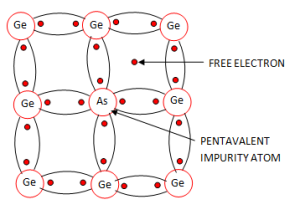Fig.2

Therefore, for each arsenic atom added, one free electron will be available in the germanium crystal.

Fig.3 shows the energy band description of n-type semiconductor.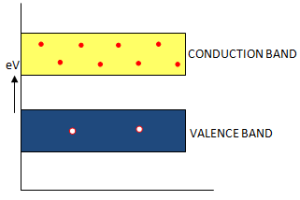Fig.3

The addition of pentavalent impurity has produced a number of conduction band electrons i.e. free electrons.

The four valence electrons of pentavalent atom form co-valent bonds with four neighbouring germanium atoms. The fifth left over valence electron of the pentavalent atom can not be accommodated in the valence band and travels to the conduction band.

The following two points may be noted carefully:

(i)   Many new free electrons are produced by the addition of pentavalent impurity.

(ii)  Thermal energy of room temperature still generated a few hole-electron pairs. However, the number of free electrons provided by the pentavalent impurity far exceeds the number of holes. Hence it is called n-type semiconductor (n stands for negative)

### n-type Conductivity

The current conduction in an n-type semiconductor is predominantly by free electrons i.e. negative charges and is called n-type or electron type conductivity.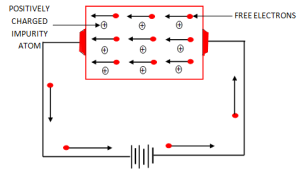Fig.4

When a potential difference is applied across the n-type semiconductor, the free electrons, donated by the impurity in the crystal, will be directed towards the positive terminal, constituting electric current.

As the current flow through the crystal is by free electrons which are carriers of negative charge, therefore, this type of conductivity is called negative or n-type conductivity.

### P-type  Semiconductor

When a small amount of trivalent impurity is added to a pure semiconductor, it is called p-type semiconductor.

The addition of trivalent impurity provides a large number of holes in the semiconductor. Typical example of trivalent impurities are gallium (Ga) and indium (In).

Such impurities which produce p-type semiconductor are known as acceptor impurities as the holes created can accept electrons.

To explain the formation of p-type semiconductor, consider a pure germanium crystal.

When a small amount of trivalent impurity like gallium is added to germanium crystal, there exist a large number of holes in the crystal. The reason is explained below:

Gallium is trivalent i.e. its atom as three valence electrons. Each atom of gallium fits into the germanium crystal but now only three co-valent bonds can be formed. It is because three valence electrons of gallium atom can form only three single co-valent bonds with three germanium atoms as shown in fig.5.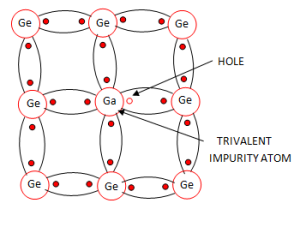Fig.5

In the fourth co-valent bond only germanium atom contributes one valence electron while gellium has no valence electron to contribute as its three valence electrons are already engaged in the co-valent bonds with neighbouring germanium atoms.

In other word, fourth bond is incomplete; being short of one electron. This missing electron is called a hole. Therefore, for each gallium atom added, one hole is created.

Fig.6 shows the energy band diagram of  p-type semiconductor.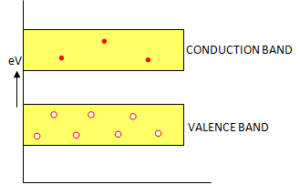Fig.6

The addition of trivalent impurity has produced a large number of holes. However, there are a few conduction band electrons due to thermal energy at room temperature. But  the number of  holes far exceeds the number of conduction band electrons.

It is due to the predominance of holes over free electrons that it is called p-type semiconductor (p stands for positive).

#### P-type Conductivity

The current conduction in p-type semiconductor is predominantly by holes i.e.  positive charges and is called p-type or hole-type conductivity.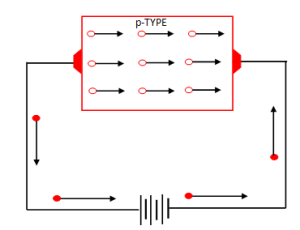Fig.7

When a potential difference is applied to the p-type semiconductor, the holes, donated by the impurity, are shifted from one co-valent bond to another.

As the holes are positively charged, therefore, they are directed towards the negative terminal, constituting hole current.

### Charges on n-type and p-type Semiconductor

In n-type semiconductor, current conduction is due to excess of electrons whereas in a p-type semiconductor, conduction is due to holes.

One may think that n-type material has a net negative charge and p-type has a net positive charge. But this conclusion is wrong. It is true that n-type semiconductor has excess of electrons but these extra electrons were supplied by the atoms of donor impurity and each atom of donor impurity is electrically neutral. When the impurity atom is added, the term excess electrons refers to an excess with regard to the number of electrons needed to fill the co-valent bonds in the semiconductor crystal. The extra electrons are free electrons and increase the conductivity of the semiconductor.

This situation with regard to p-type semiconductor is also similar.

Hence, n-type as well as p-type semiconductor is electrically neutral.

### Majority and Minority Carriers

Due to the impurity, n-type semiconductor has a large number of free electrons whereas p-type semiconductor has a large number of holes.

However, at room temperature, some of the co-valent bonds break, thus releasing equal number of  free electrons and holes.

So,an n-type semiconductor has its share of electron-hole pairs due to breaking of co-valent bonds and in addition has a much larger quantity of free electrons due to the effect of impurity. Consequently, an n-type semiconductor has a large number of free electrons and a small number of holes as shown in fig.8.Fig.8

The free electrons in this case are known as majority carriers, since the majority portion of current in n-type material is by the flow of free electrons. And the holes are known as minority carriers.

Similarly, in a p-type semiconductor, holes outnumber the free electrons as shown in fig.9.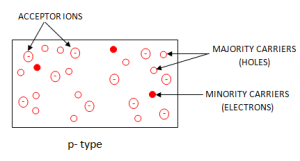Fig.9

Therefore, holes are the majority carriers and free electrons are the minority carriers.